Mobile QR Code1. ( School of Electrical and Computer Engineering, University of Seoul, Seoul, Korea)
2. ( Samsung Electronics Inc., Hwasung, Korea )

Discrete tuning, jitter, phase-locked loop, phase noise, quantization error, voltagecontrolled oscillator (VCO)

## I. INTRODUCTION

As the various chip-to-chip interface specifications become popular, researches on high-speed interface circuits are inevitable. To this end, it is important to improve both the operating frequency and low-jitter characteristics of a phase-locked loop (PLL).

Since a ring-VCO has at least three delay cells, the operating frequency of a ring-VCO is usually lower than that of a LC-VCO. The delay of a ring-VCO’s cells is controlled by not only a control voltage but also a supply voltage, thereby suffering from supply noise. For these reasons, PLLs are increasingly adopting LC-VCOs rather than ring-VCOs. However, the disadvantages of an LC-VCO are that a spiral inductor occupies large area and the capacitance of an LC-VCO should be adjusted to compensate for the (approximately 10 to 15%) process variation of the inductor. The method of adjusting the capacitance is called a discrete tuning algorithm. In conclusion, an LC-VCO needs to be employed to satisfy the high-speed operation and low-jitter characteristics, but the area of the inductor should be minimized and a precise discrete tuning algorithm should be developed.

There are roughly two types of coarse tuning algorithms. The first method adjusts the capacitor bank when a VCO control voltage (V$_{\mathrm{CTRL}}$) is outside a certain range (1,2). The other method adjusts the capacitor bank according to frequency counting while V$_{\mathrm{CTRL}}$ is fixed (3-5). Fig. 1(a) illustrates the former method and Fig. 1(b) illustrates the latter method.

Fig. 1. Coarse tuning algorithm using (a) a voltage range monitor and (b) a frequency counter.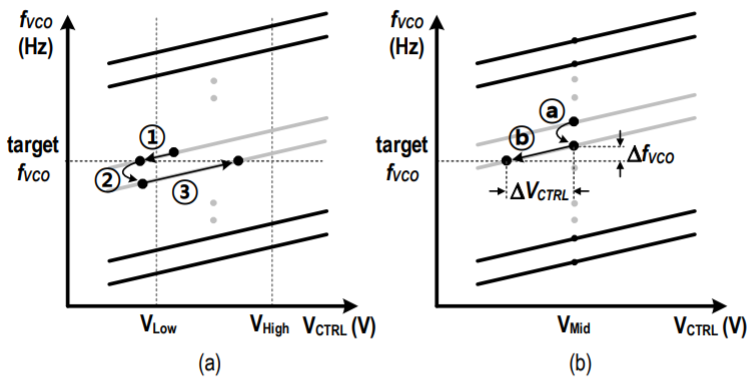Fig. 2. Comparison of the proposed discrete tuning algorithm ($CB_{P}$[5:0]) with the conventional algorithm ($CB_{C}$[5:0]). (a) The conventional algorithm and (b) the proposed algorithm with slightly larger inductance ($L = {L}_{nom}$ + $\Delta L$). (c) The conventional algorithm and (d) the proposed algorithm with slightly smaller inductance ($L = {L}_{nom}$ ${-}$ $\Delta L$)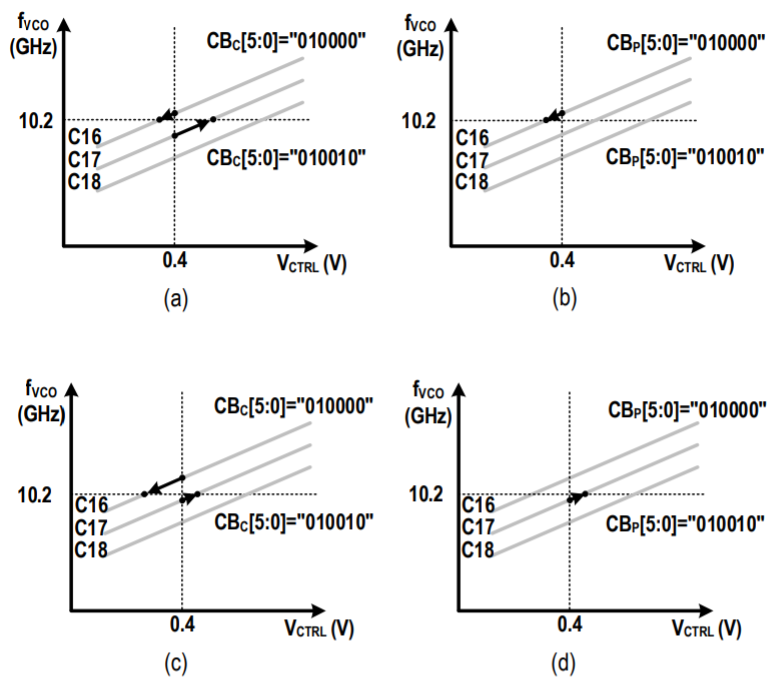Suppose that the initial V$_{\mathrm{CTRL}}$ exists slightly above V$_{\mathrm{Low}}$ as shown in Fig. 1(a). Due to voltage or temperature variation, V$_{\mathrm{CTRL}}$ can be moved to a value lower than V$_{\mathrm{Low}}$ (Process ①). The adjacent lower VCO curve is selected according to the output results of voltage comparators (Process ②). The locking operation of the PLL moves V$_{\mathrm{CTRL}}$ to a new value (Process ③).

In Fig. 1(b), V$_{\mathrm{CTRL}}$ is fixed to V$_{\mathrm{Mid}}$ during the discrete tuning process. V$_{\mathrm{Mid}}$ is determined considering the characteristics of related circuits such as the output common-mode voltage of an LC-VCO, the dynamic range of a charge pump, and the C${-}$V characteristic of a fine-tuning varactor.

A frequency counter generates UP/DN signals, which decrease/increase a capacitor-bank control signal (CB), thereby selecting a new VCO curve (Process ⓐ). The difference between the target VCO frequency (target fvco) and the selected fvco is defined as $\Delta$fvco as shown in Fig. 1(b). After VCO-curve selection is finished, V$_{\mathrm{CTRL}}$ is released and the fine tuning process begins. And then the PLL locks to the reference clock by means of control V$_{\mathrm{CTRL}}$ (Process ⓑ ). During the fine-tuning process, V$_{\mathrm{CTRL}}$ is approaching to the target V$_{\mathrm{CTRL}}$ . The difference of this target V$_{\mathrm{CTRL}}$ to V$_{\mathrm{Mid}}$ is defined as $\Delta$ V$_{\mathrm{CTRL}}$ . According to the definition of VCO gain ($K_{VCO}$ ), the following relationship is established between $\Delta$ V$_{\mathrm{CTRL}}$ and $\Delta$fvco. It is desirable that |$\Delta$ V$_{\mathrm{CTRL}}$ | and |$\Delta$fvco| are both minimized for best circuit performance.

##### (1)
\begin{equation} \tag{1} K_{VCO}\times \Delta V_{CTRL}=\Delta fvco \end{equation}

We have improved the latter method and suggest a more precise discrete tuning algorithm, which selects the nearest VCO curve to the target. Section II describes the proposed algorithm through the comparison with a conventional algorithm. Section III describes the PLL design based on an LC-VCO. Section IV describes the performance measurement results of the PLL. And finally, Section V concludes this paper.

## II. DISCRETE TUNING ALGORITHM

### 1. Conventional Algorithm vs Proposed Algorithm

Assume slightly larger inductance ($L=L_{\mathrm{nom}}$ + $\Delta L$ ) for Fig. 2(a-b) and slightly smaller inductance ($L=L_{\mathrm{nom}}$ ${-}$ $\Delta L$ ) for Fig. 2(c-d). Fig. 2(a) and (c) illustrate the conventional method while Fig. 2(b) and (d) illustrate the proposed method. $CB_{C}$[5:0] denotes a capacitor-bank control signal (CB) for the conventional method while $CB_{P}$[5:0] denotes CB for the proposed method. It is assumed that the target fvco is 10.2 GHz and V$_{\mathrm{Mid}}$ is 0.4 V.

In Fig. 2(a-b), it is assumed that the target VCO frequency exists between C16 (the VCO curve when CB[5:0] = “010000”) and C17 (the VCO curve when CB[5:0] = “010001”). |$\Delta$fvco$_{\mathrm{@C16}}$| < |$\Delta$f$_{\mathrm{VCO@C17}}$| so the nearest VCO curve is C16. $\Delta$fvco$_{\mathrm{@C16}}$ stands for $\Delta$fvco in case that C16 is selected. $\Delta$fvco$_{\mathrm{@C17}}$ stands for $\Delta$fvco in case that C17 is selected.

In Fig. 2(a), the conventional method randomly selects either C16 or C17. In Fig. 2(b), C16 is selected. Therefore, |$\Delta$fvco| in Fig. 2(b) is equal to or smaller than |$\Delta$fvco| in Fig. 2(a).

In Fig. 2(c-d), all the VCO curves are shifted up due to slight inductance reduction ($L=L_{\mathrm{nom}} - \Delta L$), resulting to |$\Delta$fvco$_{\mathrm{@C16}}$| > |$\Delta$fvco$_{\mathrm{@C17}}$|. In Fig. 2(c), either C16 or C17 is randomly selected. In Fig. 2(d), C17 is selected. Therefore, |$\Delta$fvco| in Fig. 2(d) is equal to or smaller than |$\Delta$fvco| in Fig. 2(c).

Fig. 3. Comparison of the proposed discrete tuning algorithm ($CB_{P}$[5:1] ) with the conventional algorithm ($CB_{C}$[5:0]). (a) The conventional algorithm and (b) the proposed algorithm with slightly larger inductance ($L = {L}_{nom}$ + $\Delta L$). (c) The conventional algorithm and (d) the proposed algorithm with slightly smaller inductance ($L = {L}_{nom}$ ${-}$ $\Delta L$).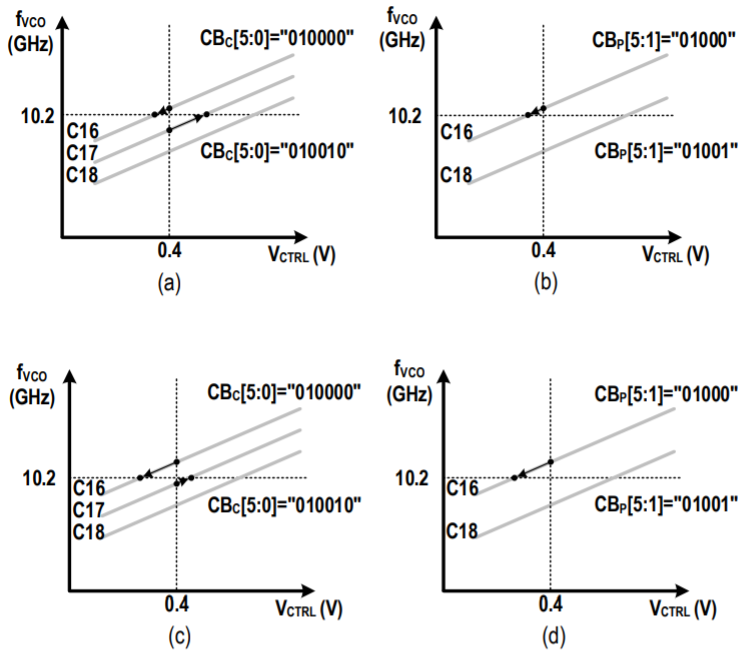The shift-up or shift-down of the VCO curves can be caused by not only the inductance variation but also other process, voltage, and temperature (PVT) variations. In conclusion, even if the VCO curves are shifted according to PVT variations including the inductance variation, |$\Delta$fvco| of the proposed method is equal to or smaller than |$\Delta$fvco| of the conventional method. As shown in Fig. 1(b) and the equation (1), as |$\Delta$fvco| decreases, so does |$\Delta$V$_{CTRL}$|. This means that $V_{CTRL}$ gets closer to VMid. Therefore, as mentioned above, the proposed method shows better circuit performance than that of the conventional method. In other words, the proposed method using the same bit number of a capacitor-bank control signal ($CB_{P}$[5:0]) will have additional 1-bit resolution. Fig. 3 describes this in detail.

### 2. Conventional Algorithm with $CB_{C}$[5:0] vs Proposed Algorithm with $CB_{P}$[5:1]

In Fig. 3, it is assumed that the conventional method uses $CB_{C}$[5:0] and the proposed method uses $CB_{P}$[5:1] . Fig. 3(a) and (c) are the same as Fig. 2(a) and (c), respectively. Fig. 3(b) and (d) shows the proposed method using $CB_{P}$[5:1] . A major difference of the proposed method compared to the conventional method is that every other curve disappears by removing the LSB ($CB_{P}$) of $CB_{P}$[5:0].

Fig. 4. Input/output characteristic of the conventional and the proposed discrete tuning algorithms.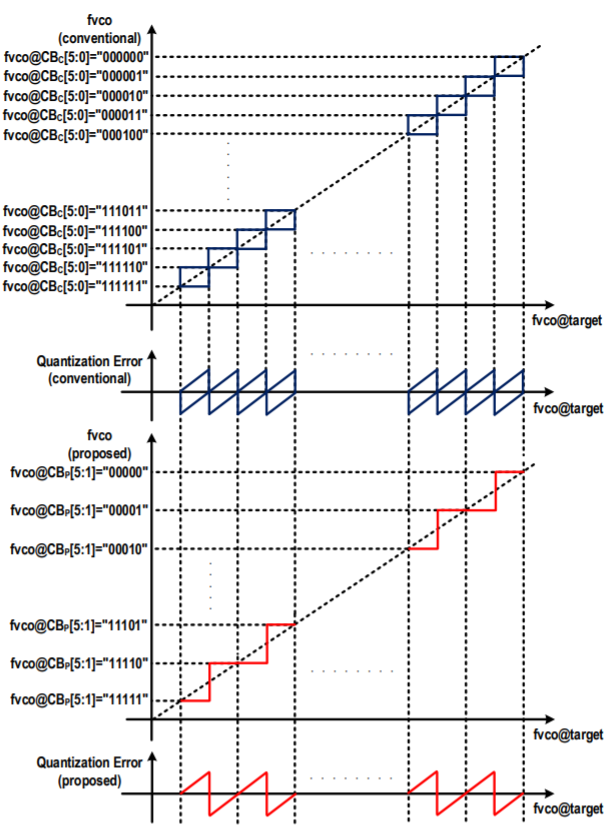In Fig. 3(b), C16 is selected, leading to $\Delta$fvco$_{\mathrm{@C16}}$. Therefore, |$\Delta$fvco| in Fig. 3(b) is equal to or smaller than |$\Delta$fvco| in Fig. 3(a). In Fig. 3(d), C16 is selected, leading to $\Delta$fvco$_{\mathrm{@C16}}$. Therefore, |$\Delta$fvco| in Fig. 3(d) is equal to or greater than |$\Delta$fvco| in Fig. 3(c).

Fig. 4 shows the input/output characteristics of the discrete tuning algorithms with CB. The fvco on the x-axis means the target fvco and the fvco on the y-axis means the fvco selected right after discrete tuning. Quantization error on the y-axis means the difference ($\Delta$fvco) between the target fvco and the selected fvco. In the conventional method, quantization error can have two values because either of the two VCO curves is selected randomly.

However, in the proposed method, quantization error has only one value because the nearest VCO curve is selected. Fig. 5(a-b) again show quantization errors of the conventional algorithm and the proposed algorithm respectively. As shown in the following formula, the mean square value ($\overline{\mathcal{ε}^{2}}$) of quantization error (ε) is derived in the same way for both the conventional and the proposed algorithms. Despite a 1-bit reduction in LSB of the CB control signal, the proposed method has the same ($\overline{\mathcal{ε}^{2}}$) as the conventional method.

Fig. 5. Quantization errors of the conventional and the proposed discrete tuning algorithms.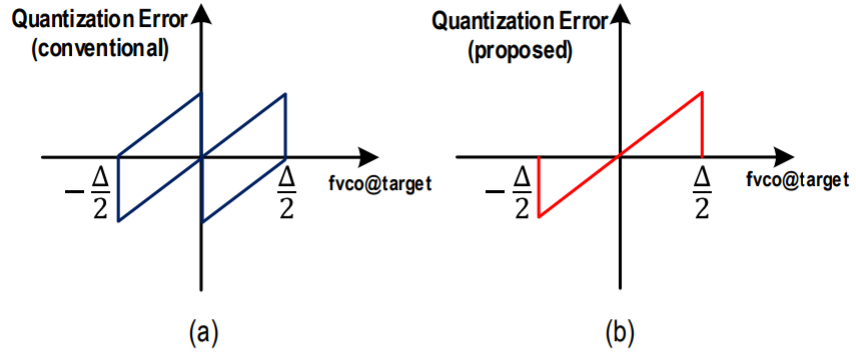##### (2)
$$\overline{\varepsilon^{2}}=\frac{1}{\Delta} \int_{-\frac{4}{2}}^{\frac{\Delta}{2}} \varepsilon^{2} d \varepsilon=\frac{\Delta^{2}}{12}$$

From the point of view of circuit design, the operating frequency of the LC-VCO can increase slightly because the parasitic capacitance corresponding to LSB (CB) is removed. And the design of the capacitor bank can be easier since the metal capacitor for the LSB has about 9.5 fF and its size is too small to be supported by process design kits (PDKs).

## III. PLL DESIGN BASED ON LC-VCO

The designed LC-VCO circuit is shown in Fig. 6. A 5-bit cap bank is incorporated for the discrete tuning of the VCO frequency and varactors are used for fine tuning. A Gm-cell consists of cross-coupled NMOS and PMOS pairs, replenishing the energy lost by the parasitic resistance of the inductor, the cap bank, and the varactors. As shown in Fig. 7, the LC-VCO exhibits thirty two curves with CB[5:1].

The Gm-cell is biased by a top-current source because the top-current structure has higher supply-noise immunity than the bottom-current structure. Since the bias circuit for the LC-VCO is designed to maintain the value of ‘V$_{DD}$ - V$_{pbias}$’, the current of the current source rarely varies even if supply noise occurs and thus the DC value of LC-VCO’s outputs is not significantly changed. As a result, $\frac{\partial f_{v c o}}{\partial V D D}$ VDD is minimized.

Fig. 8 is the block diagram of the overall PLL. The LC-VCO generates 10.2-GHz clocks and delivers them to a current-mode-logic divide-by-2 circuit (CML DIV2) to create 5.1-GHz clocks (PLL_CLK). In order to reduce the size of the inductor, 10.2-GHz frequency is created instead of 5.1-GHz frequency. PLL_CLK is divided by 30 to be a 170-MHz clock (PCLK170X), which is connected to an input signal (PCLK) of Frequency Counter. REF_CLK (85 MHz) is divided by 200, generating REFCLK_N (425 kHz).

Fig. 9 is the block diagram of Frequency Counter. The clock cycles of PCLK are counted to be CNT2. REFCLK_N samples CNT2 to generate CNT1 and delays CNT1 to create CNT0. INC1 (= CNT1 - CNT0) corresponds to the Counter value increased during one period of REFCLK_N. INC1 is compared with a threshold value K (= 400) to determine how high or low the frequency of PCLK is (DIFF1 = INC1 - K).

CB_Control in Fig. 8 receives UP_DN1, UP_DN0, and GRAVITY1 signals, thereby generating CB[5:1] and FREEZE. During the discrete tuning process, CB_Control keeps FREEZE at “1” to hold $V_{CTRL}$.

The proposed discrete tuning algorithm consists of binary search and then linear search. During the binary search process, CB to CB are sequentially determined, which is similar to the methods presented in (6-7). Assume the relationship between CB[5:1] and VCO curves as shown in Fig. 3(b), Fig. 3(d), and Fig. 10. CB[5:1] is “10000 (C32)” in the first REFCLK_N period (T1 period), “01000 (C16)” in T2 period, “01100 (C24)” in T3 period, “01010 (C20)” in T4 period, “01001 (C18)” in T5 period, and “01000 (C16)” in T6 period.

Afterwards, if the direction of VCO-curve selection is changed in the linear search step, the optimal value is selected between the CB values for the current and previous two periods and the discrete tuning process is finished. The optimal value corresponds to the nearest VCO curve.

Fig. 10 illustrates an example timing diagram of the Discrete Control block. Suppose the LC-VCO frequency (fvco) in T5 period is 10.12 GHz, which is lower than the ideal value (10.2 GHz). In T6 period, INC1 = 397 and DIFF1 = 3. DIFF1 is negative and UP_DN1 is ‘0’ to decrease CB[5:1], thereby increasing fvco. DIFF1 is one-period delayed to generate DIFF0. GRAVITY1 is generated using the sign of the sum (MASS1) of DIFF1 and DIFF0.

If UP_DN1 is different from UP_DN0, it indicates that the direction of VCO-curve selection is changed and the discrete tuning process has been completed. Therefore, FREEZE is set to be ‘0’ as shown in T7 period.

In the end of the discrete tuning process, GRAVITY1 determines the final value of CB[5:1]. As shown in Fig. 10, GRAVITY1 is ‘0’ in T7 period so a lower value (“01000”) is chosen between CB[5:1] in T6 period and CB[5:1] in T5 period. If GRAVITY1 = ‘1’, a larger value (“01001”) would have been chosen.

## IV. EXPERIMENTAL RESULTS

The PLL with a Discrete Control block is designed using a 28-nm CMOS process. A serializer (P2S) and a driver are incorporated to monitor a PLL’s clock signal. Fig. 11 shows the layout of the PLL, whose area is 324 μm × 432 μm. The layout size of the inductor is 169 $\mu$m × 169 $\mu$m. The S-parameter of the inductor is extracted using EMX tool and post-layout simulations are performed.

Fig. 12 shows a photograph of the chip. For the impedance matching, both transmission lines of the test board and the output impedance of the driver are designed to have 50 ohms.

Fig. 13 shows a measurement environment. JBERT provides a trigger clock to the sampling scope and an 85-MHz reference clock (Ref_Clk) to the device under test (DUT). As shown in Fig. 8, the serializer (P2S) in the DUT was preset to generate a clock ("1010") pattern. Using the differential signals (TXP and TXN) as inputs of a sampling scope and a spectrum analyzer, jitter, phase noise, and frequency spectrum are measured.

Fig. 14 shows the measured spectrum of the transmitted PLL clock in the free-running mode. For this mode, CB[5:1] is forced to the desired value and FREEZE is forced to '1' by means of setting TEST_EN = '1' as shown in Fig. 8. Fig. 14(a) shows that the transmitted PLL clock has 5.07 GHz when CB[5:1] = "01001". Fig. 14(b) shows that it has 5.12 GHz when CB[5:1] = "01000".

Fig. 14. Measured spectrum of the transmitted PLL clock in free running mode (a) when CB[5:1] = “01001” (5.07 GHz) and (b) when CB[5:1] = “01000” (5.12 GHz).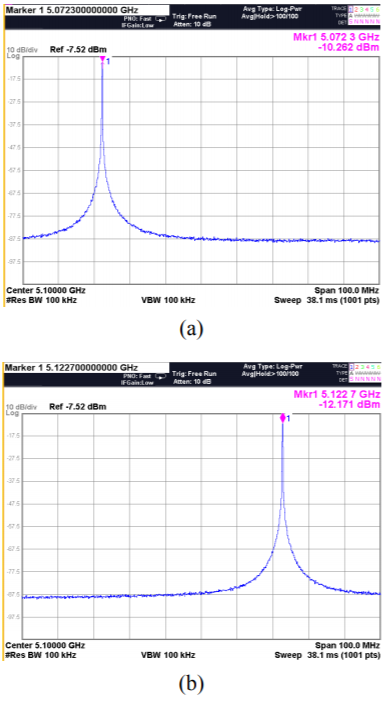Fig. 15. Measured spectrum of the transmitted PLL clock in reference-locking mode with (a) 100-MHz span and (b) 10-MHz span.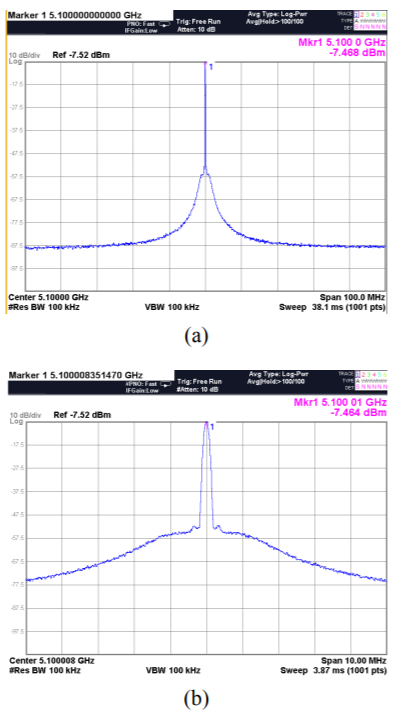Fig. 16. Measured phase noise of (a) the reference clock and (b) the transmitted PLL clock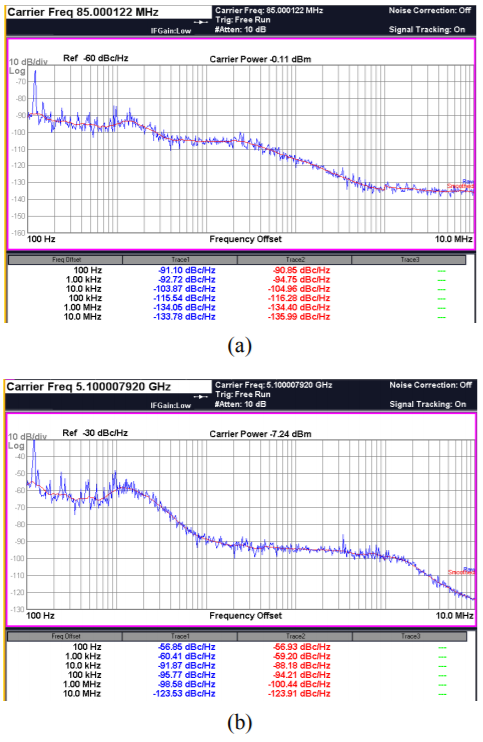Fig. 15 shows the measured spectrum when the PLL locks to the reference clock, exhibiting 5.1 GHz. Because the Discrete Control block selects the VCO curve closest to the target during the discrete tuning process, it is expected that CB[5:1] was "01000" as shown in Fig. 14(b). In the free-running mode, as shown in Fig. 14(a-b), there is significant VCO phase noise near the center frequency. However in the reference-locking mode (Fig. 15), the VCO phase noise is quite suppressed.

Fig. 16 shows the measured phase noise. The phase noise of the reference clock is -116.28 dBc/Hz at a frequency offset of 100 kHz and the phase noise of the transmitted PLL clock is -94.21 dBc/Hz at a frequency offset of 100 kHz.

Fig. 17 shows the output waveforms and jitter characteristics measured by the oscilloscope. For jitter analysis a built-in software in the oscilloscope is used. In measuring jitter, the hit number is approximately 10k. The jitter of the reference clock is as follows. RJ (Random Jitter) = 1 ps, DJ (Deterministic Jitter) = 0 ps, and TJ (Total Jitter) = 13.6 ps. The jitter of the transmitted PLL clock is as follows. RJ = 1.29 ps, DJ = 2.3 ps, and TJ = 20.1 ps.

Fig. 17. Using a built-in jitter analysis software, measured waveforms and jitter characteristics of (a) the reference clock and (b) the transmitted PLL clock.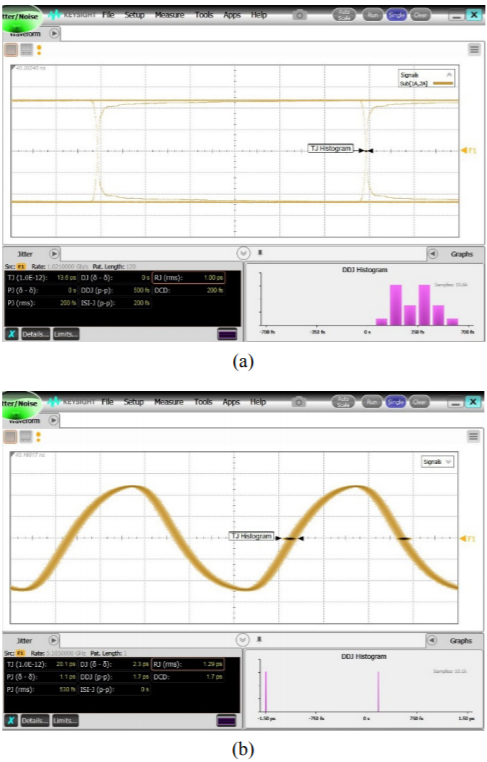Suppose that $\sigma$(x) is the RMS jitter of the reference clock and $\sigma$(y) is the RMS jitter of the PLL itself, where $\sigma$(x + y) may correspond to the RMS jitter of the transmitted PLL clock in Fig. 17(b). If we can use $\sigma^{2}$2(x + y) = $\sigma^{2}$2(x) + $\sigma^{2}$2(y), where $\sigma$(x + y) = 1.29 ps and $\sigma$(x) = 1 ps, the RMS jitter of the PLL itself is approximately 815 fs.

Fig. 18 represents the jitter histogram of the transmitted PLL clock. The measured jitter is 1.55-ps RMS and 12.1-ps peak-to-peak. Under the same measurement condition, the reference clock jitter is 1.24-ps RMS and 9.8-ps peak-to-peak.

Table 1 summarizes the measured performance of the test chip. The driver has power consumption of 23 mW with a 1-V supply at 10.2-GHz LC-VCO frequency. The PLL incorporating the LC-VCO consumes 3 mW at the same condition.

Fig. 18. Jitter histogram of the transmitted PLL clock in the reference-locking mode.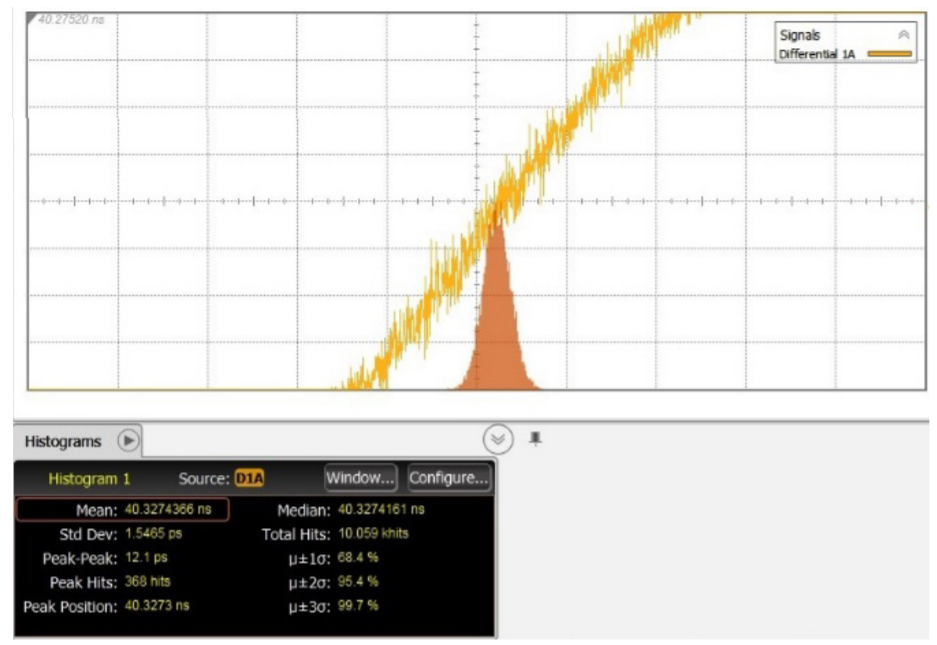## V. CONCLUSIONS

An 8.48-to-11.13 GHz LC-VCO is designed for a PLL. For the discrete tuning of the LC-VCO, a new algorithm to select the VCO curve closest to the target frequency is proposed, which exhibits additional 1-bit resolution. The proposed algorithm even with the LSB of the capacitor-bank control signal being removed shows the same level of quantization error as the conventional algorithm. The PLL is implemented using a 28-nm CMOS process and it occupies 0.14 mm$^{2}$. The transmitted PLL clock has a phase noise of -94.21 dBc/Hz at 100-kHz offset and the jitter of

815-fs RMS can be obtained by de-embedding the jitter of the reference clock. The PLL and the driver have power consumption of 3 mW and 23 mW respectively with a 1-V supply at 10.2-GHz LC-VCO frequency.

### ACKNOWLEDGMENTS

This research was supported by the MOTIE(Ministry of Trade, Industry & Energy (10080285) and KSRC(Korea Semiconductor Research Consortium) support program for the development of the future semiconductor device.

This paper is supported by Future Interconnect Technology Cluster Program of Samsung Electronics.

### REFERENCES

1
Lee H.-R., Hwang M.-S., Lee B.-J., Kim Y.-D., Oh D., Kim J., Lee S.-H., Jeong D.-K., Kim W., Nov 2005, A 1.2-V-Only 900-mW 10 Gb Ethernet Transceiver and XAUI Interface With Robust VCO Tuning Technique, IEEE J. Solid-State Circuits, Vol. 40, No. 11, pp. 2148-21582
Lin T.-H., Kaiser W. J., Mar 2001, A 900-MHz 2.5-mA CMOS frequency synthesizer with an automatic SC tuning loop, IEEE J. Solid-State Circuits, Vol. 36, No. 3, pp. 424-4313
Ravi A., Banerjee G., Bishop R. E., Bloechel B. A., Carley L. R., Soumyanath K., Jun 2003, 10 GHz, 20 mW, fast locking, adaptive gain PLLs with on-chip frequency calibration for agile frequency synthesis in a 0.18 m digital CMOS process, in Symp. VLSI Circuits Dig. Tech. Papers, pp. 181-1844
Wilson W. B., Moon U.-K., Lakshmikumar K. R., Dai L., Oct 2000, A CMOS self-calibrating frequency synthesizer, IEEE J. Solid-State Circuits, Vol. 35, No. 10, pp. 1437-14445
Pottbacker A., Langmann U., Schreiber H. U., Dec 1992, A Si bipolar phase and frequency detector IC for clock extraction up to 8 Gb/s, IEEE J. Solid-State Circuits, Vol. 27, No. 12, pp. 1747-17516
Lee H.-I., Cho J.-K., Lee K.-S., Hwang I.-C., Ahn T.-W., Nah K.-S., Park B.-H., Jul 2004, A $\Sigma-\Delta$ Fractional-N Frequency Synthesizer Using a Wide-Band Integrated VCO and a Fast AFC Techniques for GSM/GPRS/WCDMA Applications, IEEE J. Solid-State Circuits, Vol. 39, No. 7, pp. 1164-11697
Lee C.-H., Ali A., Lloyd S., 2004, A 0.18um SiGe BiCMOS UHF VCO with Auto Tuning for DCT AMPS and CDMA Application, in Dig. Papers, IEEE Radio Freq. Integr. Circuits Symp, pp. 471-474## Author

Myung-Hun Jung was born in Gyeonggi-do, Korea, in 1990.

He received the B. S. and the M. S. degrees in the School of Electrical and Computer Engineering, University of Seoul, Seoul, Korea, in 2018 and 2020, respectively. In 2020, he joined Samsung Electronics, Hwasung, Korea.

His current research interests include phase-locked loop and LC-VCO circuits for high-speed communication and high-speed I/O interface circuits.

Hyeon-Jin Yang was born in Yecheon, Korea, in 1995.

He received the B.S. degree in the School of Electrical and Computer Engineering, University of Seoul, Seoul, Korea, in 2018.

He is currently working toward the M. S. degree at the same university.

His research interests include clock and data recovery for high-speed communication and high-speed I/O interface circuits.

Hye-Seong Shin was born in Incheon, Korea, in 1993.

He received the B. S. degree in the School of Electrical and Computer Engineering, University of Seoul, Seoul, Korea, in 2019.

He is currently working toward the M.S. degree at the same university.

His current research interests include clock and data recovery for high-speed communication and high-speed I/O interface circuits.

Young-Gil Go was born in Sokcho, Korea, in 1994.

He received the B.S degrees in the School of Electrical and Computer Engineering, University of Seoul, Seoul, Korea, in 2019.

He is currently working toward the M. S. degree at the same university.

His research interest include clock and data recovery for high-speed communication and high-speed I/O interface circuits.

Jung-Sik Kim was born in Seoul, Korea.

He received the B.S. and M.S. degrees in the School of Electrical and Computer Engineering, University of Seoul, Seoul, Korea, in 2017 and 2019, respectively.

In 2019, he joined Samsung Electronics, Hwasung, Korea.

His interest includes LC voltage-controlled oscillators and phase locked loop circuits.